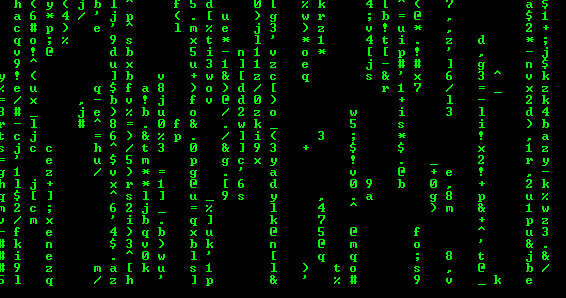# Implementation of a Falling Matrix

Since the dawn of computers, Hollywood has greatly demonstrated a Hacker or a Programmer as someone sitting on a computer typing random keys on computer which ultimately compiles to a Falling matrix like simulation. Here, we will try to implement a similar falling matrix simulation on the console using C++.The idea here is to print random characters over a defined width, where the two successive characters may or may not have certain amount of gap defined randomly. A certain amount of delay between printing successive lines has to be implemented in order to have a ‘falling effect’.

## Recommended: Please try your approach on {IDE} first, before moving on to the solution.

 `// C++ program for implementation of falling matrix. ` `#include ` `#include ` `#include ` `#include ` `#include ` `#include ` ` `  `// Width of the matrix line ` `const` `int` `width = 70; ` ` `  `// Defines the number of flips in Boolean Array 'switches' ` `const` `int` `flipsPerLine =5; ` ` `  `// Delay between two successive line print ` `const` `int` `sleepTime = 100; ` ` `  `using` `namespace` `std; ` ` `  `int` `main() ` `{ ` `    ``int` `i=0, x=0; ` ` `  `    ``// srand initialized with time function ` `    ``// to get distinct rand values at runtime ` `    ``srand``(``time``(NULL)); ` ` `  `    ``// Used to decide whether to print ` `    ``// the character in that particular iteration ` `    ``bool` `switches[width] = {0}; ` ` `  `    ``// Set of characters to print from ` `    ``const` `string ch = ``"1234567890qwertyuiopasdfghjkl"` `                      ``"zxcvbnm,./';[]!@#\$%^&*()-=_+"``; ` `    ``const` `int` `l = ch.size(); ` ` `  `    ``// Green font over black console, duh! ` `    ``system``(``"Color 0A"``); ` ` `  `    ``// Indefinite Loop ` `    ``while` `(``true``) ` `    ``{ ` `        ``// Loop over the width ` `        ``// Increment by 2 gives better effect ` `        ``for` `(i=0;i

This prints the amazing Falling-Matrix simulation on the console.

Note :

• This program would not run using Run on IDE button because system is disabled.
• If you get compiler error while compiling this program. Compile it using below command on GCC.
```\$ g++ -std=c++11 abc.cpp -o falling.o
\$ falling.o ```

This article is contributed by Raghav Jajodia. If you like GeeksforGeeks and would like to contribute, you can also write an article using contribute.geeksforgeeks.org or mail your article to contribute@geeksforgeeks.org. See your article appearing on the GeeksforGeeks main page and help other Geeks.